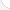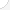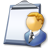Drafters Description: Drafters prepare the drawings used to build everything from spacecraft to bridges. Using rough sketches done by others, they produce detailed technical drawings with specific information to create a finished product. Drafters use handbooks, tables, calculators, and computers to do their work. Many specialize in architecture, electronics, or aeronautics.Source: Bureau of Labor Statistics, U.S. Department of Labor, Occupational Outlook Handbook, 2008-09 Edition at http://www.bls.gov/OCO/Complete Job Profile: http://www.bls.gov/oco/ocos111.htmSalary: \$28,001 to \$50,000 per yearComments:

There are 38 math topics Drafters need to know.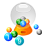Basic Math / Algebra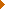DecimalsRatio and ProportionCustomary MeasurementMetric MeasurementMeasurement ConversionPowers and RootsNegative NumbersScientific NotationBasic Problem Solving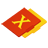First-Year AlgebraUsing FormulasLinear EquationsCoordinate Graphing 2D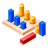GeometryBasic TerminologyAngle MeasurementCongruent TrianglesTriangle InequalitiesParallel LinesQuadrilateralsSimilarityPythagorean TheoremRight Triangle TrigonometryCirclesConstructionsAreaTransformationsMake/Use 3D Drawings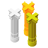Second-Year Algebra / TrigonometryCoordinate Graphing 3DConic SectionsTrigonometric/Circular FunctionsOblique TrianglesPolar Coordinates/GraphsVectors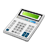Other TopicsBasic Calculator UseScientific Calculator UseComputer UseGroup Problem SolvingMental MathMath Communications